# The FREQ Procedure

### EXACT Statement

Subsections:

• EXACT statistic-options < / computation-options > ;

The EXACT statement requests exact tests and confidence limits for selected statistics. The statistic-options identify which statistics to compute, and the computation-options specify options for computing exact statistics. For more information, see the section Exact Statistics.

Note: PROC FREQ computes exact tests by using fast and efficient algorithms that are superior to direct enumeration. Exact tests are appropriate when a data set is small, sparse, skewed, or heavily tied. For some large problems, computation of exact tests might require a considerable amount of time and memory. Consider using asymptotic tests for such problems. Alternatively, when asymptotic methods might not be sufficient for such large problems, consider using Monte Carlo estimation of exact p-values. You can request Monte Carlo estimation by specifying the MC computation-option in the EXACT statement. See the section Computational Resources for more information.

#### Statistic Options

The statistic-options specify which exact tests and confidence limits to compute. Table 40.6 lists the available statistic-options and the exact statistics that are computed. Descriptions of the statistic-options follow the table in alphabetical order.

For one-way tables, exact p-values are available for binomial proportion tests, the chi-square goodness-of-fit test, and the likelihood ratio chi-square test. Exact (Clopper-Pearson) confidence limits are available for the binomial proportion.

For two-way tables, exact p-values are available for the following tests: Pearson chi-square test, likelihood ratio chi-square test, Mantel-Haenszel chi-square test, Fisher’s exact test, Jonckheere-Terpstra test, and Cochran-Armitage test for trend. Exact p-values are also available for tests of the following statistics: Pearson correlation coefficient, Spearman correlation coefficient, Kendall’s tau-b, Stuart’s tau-c, Somers’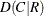, Somers’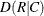, simple kappa coefficient, and weighted kappa coefficient.

Fortables, PROC FREQ provides the exact McNemar’s test, exact confidence limits for the odds ratio, and Barnard’s unconditional exact test for the risk (proportion) difference. PROC FREQ also provides exact unconditional confidence limits for the risk difference and for the relative risk (ratio of proportions). For stratifiedtables, PROC FREQ provides Zelen’s exact test for equal odds ratios, exact confidence limits for the common odds ratio, and an exact test for the common odds ratio.

Most of the statistic-option names listed in Table 40.6 are identical to the corresponding option names in the TABLES and OUTPUT statements. You can request exact computations for groups of statistics by using statistic-options that are identical to the TABLES statement options CHISQ , MEASURES , and AGREE . For example, when you specify the CHISQ statistic-option in the EXACT statement, PROC FREQ computes exact p-values for the Pearson chi-square, likelihood ratio chi-square, and Mantel-Haenszel chi-square tests for two-way tables. You can request an exact test for an individual statistic by specifying the corresponding statistic-option from the list in Table 40.6.

##### Using the EXACT Statement with the TABLES Statement

You must use a TABLES statement with the EXACT statement. If you use only one TABLES statement, you do not need to specify the same options in both the TABLES and EXACT statements; when you specify a statistic-option in the EXACT statement, PROC FREQ automatically invokes the corresponding TABLES statement option. However, when you use an EXACT statement with multiple TABLES statements, you must specify options in the TABLES statements to request statistics. PROC FREQ then provides exact tests or confidence limits for those statistics that you also specify in the EXACT statement.

Table 40.6: EXACT Statement Statistic Options

Statistic Option

Exact Statistics

McNemar’s test (fortables), simple kappa test,

weighted kappa test

Barnard’s test (fortables)

Binomial proportion tests for one-way tables

Chi-square goodness-of-fit test for one-way tables;

Pearson chi-square, likelihood ratio chi-square, and

Mantel-Haenszel chi-square tests for two-way tables

Confidence limits for the common odds ratio,

common odds ratio test (for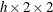tables)

Zelen’s test for equal odds ratios (fortables)

Fisher’s exact test

Jonckheere-Terpstra test

Test for the simple kappa coefficient

Test for Kendall’s tau-b

Likelihood ratio chi-square test (one-way and two-way tables)

McNemar’s test (fortables)

Tests for the Pearson correlation and Spearman correlation,

confidence limits for the odds ratio (fortables)

Mantel-Haenszel chi-square test

Confidence limits for the odds ratio (fortables)

Pearson chi-square test (one-way and two-way tables)

Test for the Pearson correlation coefficient

Confidence limits for the relative risk (fortables)

Confidence limits for the proportion difference (fortables)

Test for the Spearman correlation coefficient

Test for Somers’Test for Somers’Test for Stuart’s tau-c

Cochran-Armitage test for trend

Test for the weighted kappa coefficient

You can specify the following statistic-options:

AGREE

requests McNemar’s exact test, an exact test for the simple kappa coefficient, and an exact test for the weighted kappa coefficient. For more information, see the sections Tests and Measures of Agreement and Exact Statistics.

For McNemar’s test, you can specify the null hypothesis ratio of discordant proportions by using the AGREE(MNULLRATIO=) option in the TABLES statement; by default, MNULLRATIO=1. For the weighted kappa coefficient, you can request Fleiss-Cohen weights by specifying the AGREE(WT=FC) option in the TABLES statement; by default, PROC FREQ computes the weighted kappa coefficient by using Cicchetti-Allison agreement weights.

McNemar’s test is available fortables. Kappa coefficients are defined only for square two-way tables, where the number of rows equals the number of columns. If your table is not square because some observations have weights of 0, you can specify the ZEROS option in the WEIGHT statement to include these observations in the analysis. For more information, see the section Tables with Zero-Weight Rows or Columns.

Fortables, the weighted kappa coefficient is equivalent to the simple kappa coefficient, and PROC FREQ displays only analyses for the simple kappa coefficient.

BARNARD

requests Barnard’s exact unconditional test for the risk (proportion) difference fortables. For more information, see the section Barnard’s Unconditional Exact Test.

To request exact unconditional confidence limits for the risk difference. you can specify the RISKDIFF option in the EXACT statement. The RISKDIFF option in the TABLES statement provides asymptotic tests and several types of confidence limits for the risk difference. For more information, see the section Risks and Risk Differences.

BINOMIAL
BIN

requests an exact test for the binomial proportion (for one-way tables). For more information, see the section Binomial Tests. You can specify the null hypothesis proportion by using the BINOMIAL(P=) option in the TABLES statement; by default, P=0.5.

The BINOMIAL option in the TABLES statement provides exact (Clopper-Pearson) confidence limits for the binomial proportion by default. You can specify the BINOMIAL(CL=MIDP) option in the TABLES statement to request exact mid-p confidence limits for the binomial proportion. The BINOMIAL option in the TABLES statement also provides asymptotic (Wald) tests and several other confidence limit types for the binomial proportion. For more information, see the section Binomial Proportion.

CHISQ

requests the following exact chi-square tests for two-way tables: Pearson chi-square, likelihood ratio chi-square, and Mantel-Haenszel chi-square. For more information, see the section Chi-Square Tests and Statistics. The CHISQ option in the TABLES statement provides asymptotic tests for these statistics.

For one-way tables, the CHISQ option requests an exact chi-square goodness-of-fit test. You can specify null hypothesis proportions for this test by using the CHISQ(TESTP=) option in the TABLES statement. By default, the one-way chi-square test is based on the null hypothesis of equal proportions. For more information, see the section Chi-Square Test for One-Way Tables.

COMOR

requests an exact test and exact confidence limits for the common odds ratio for multiwaytables. For more information, see the section Exact Confidence Limits for the Common Odds Ratio. The CMH option in the TABLES statement provides Mantel-Haenszel and logit estimates of the common odds ratio along with their asymptotic confidence limits.

EQOR
ZELEN

requests Zelen’s exact test for equal odds ratios for multiwaytables. For more information, see the section Zelen’s Exact Test for Equal Odds Ratios. The CMH option in the TABLES statement provides an (asymptotic) Breslow-Day test for homogeneity of odds ratios.

FISHER

requests Fisher’s exact test. For more information, see the sections Fisher’s Exact Test and Exact Statistics. Fortables, the CHISQ option in the TABLES statement provides Fisher’s exact test. For general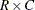tables, Fisher’s exact test is also known as the Freeman-Halton test.

JT

requests an exact Jonckheere-Terpstra test. For more information, see the sections Jonckheere-Terpstra Test and Exact Statistics. The JT option in the TABLES statement provides an asymptotic Jonckheere-Terpstra test.

KAPPA

requests an exact test for the simple kappa coefficient. For more information, see the sections Simple Kappa Coefficient and Exact Statistics. The AGREE option in the TABLES statement provides the simple kappa estimate, standard error, and confidence limits. The KAPPA option in the TEST statement provides an asymptotic test for the simple kappa coefficient.

Kappa coefficients are defined only for square two-way tables, where the number of rows equals the number of columns. If your table is not square because some observations have weights of 0, you can specify the ZEROS option in the WEIGHT statement to include these observations in the analysis. For more information, see the section Tables with Zero-Weight Rows or Columns.

KENTB
TAUB

requests an exact test for Kendall’s tau-b. For more information, see the sections Kendall’s Tau-b and Exact Statistics. The MEASURES option in the TABLES statement provides an estimate and standard error of Kendall’s tau-b. The KENTB option in the TEST statement provides an asymptotic test for Kendall’s tau-b.

LRCHI

requests an exact test for the likelihood ratio chi-square for two-way tables. For more information, see the sections Likelihood Ratio Chi-Square Test and Exact Statistics. The CHISQ option in the TABLES statement provides an asymptotic likelihood ratio chi-square test for two-way tables.

For one-way tables, the LRCHI option requests an exact likelihood ratio goodness-of-fit test. You can specify null hypothesis proportions by using the CHISQ(TESTP=) option in the TABLES statement. By default, the one-way test is based on the null hypothesis of equal proportions. For more information, see the section Likelihood Ratio Chi-Square Test for One-Way Tables.

MCNEM

requests an exact McNemar’s test. For more information, see the sections McNemar’s Test and Exact Statistics. You can specify the null hypothesis ratio of discordant proportions by using the AGREE(MNULLRATIO=) option in the TABLES statement; by default, MNULLRATIO=1. The AGREE option in the TABLES statement provides an asymptotic McNemar’s test.

MEASURES

requests exact tests for the Pearson and Spearman correlations. For more information, see the sections Pearson Correlation Coefficient, Spearman Rank Correlation Coefficient, and Exact Statistics. The PCORR and SCORR options in the TEST statement provide asymptotic tests for the Pearson and Spearman correlations, respectively.

The MEASURES option also requests exact confidence limits for the odds ratio fortables. For more information, see the subsection Exact Confidence Limits in the section Confidence Limits for the Odds Ratio. You can also request exact confidence limits for the odds ratio by specifying the OR option in the EXACT statement.

MHCHI

requests an exact test for the Mantel-Haenszel chi-square. For more information, see the sections Mantel-Haenszel Chi-Square Test and Exact Statistics. The CHISQ option in the TABLES statement provides an asymptotic Mantel-Haenszel chi-square test.

OR
ODDSRATIO

requests exact confidence limits for the odds ratio fortables. For more information, see the subsection "Exact Confidence Limits" in the section Confidence Limits for the Odds Ratio.

You can request exact mid-p confidence limits for the odds ratio by specifying the OR(CL=MIDP) option in the TABLES statement. The OR(CL=) option in the TABLES statement also provides other types of confidence limits for the odds ratio. For more information, see the section Confidence Limits for the Odds Ratio.

The ALPHA= option in the TABLES statement determines the confidence level of the exact confidence limits; by default, ALPHA=0.05, which produces 95% confidence limits for the odds ratio.

PCHI

requests an exact test for the Pearson chi-square for two-way tables. For more information, see the sections Pearson Chi-Square Test for Two-Way Tables and Exact Statistics. The CHISQ option in the TABLES statement provides an asymptotic Pearson chi-square test.

For one-way tables, the PCHI option requests an exact chi-square goodness-of-fit test. You can specify null hypothesis proportions by using the CHISQ(TESTP=) option in the TABLES statement. By default, the goodness-of-fit test is based on the null hypothesis of equal proportions. For more information, see the section Chi-Square Test for One-Way Tables.

PCORR

requests an exact test for the Pearson correlation coefficient. For more information, see the sections Pearson Correlation Coefficient and Exact Statistics. The MEASURES option in the TABLES statement provides the estimate and standard error of the Pearson correlation. The PCORR option in the TEST statement provides an asymptotic test for the Pearson correlation.

RELRISK < (options)>

requests exact unconditional confidence limits for the relative risk fortables. PROC FREQ computes the confidence limits by inverting two separate one-sided exact tests (Santner and Snell 1980). By default, this computation uses the unstandardized relative risk as the test statistic. If you specify the RELRISK(METHOD=SCORE) option, the computation uses the score statistic (Chan and Zhang 1999). For more information, see the subsection "Exact Unconditional Confidence Limits" in the section Confidence Limits for the Relative Risk.

The RELRISK(CL=) option in the TABLES statement provides additional types of confidence limits for the relative risk. For more information, see the section Confidence Limits for the Risk Difference.

The ALPHA= option in the TABLES statement determines the confidence level of the exact confidence limits; by default, ALPHA=0.05, which produces 95% confidence limits for the relative risk.

You can specify the following options:

COLUMN=1 | 2 | BOTH

specifies the table column for which to compute the relative risk. The default is COLUMN=1, which provides exact confidence limits for the column 1 relative risk. If you specify COLUMN=BOTH, PROC FREQ provides exact confidence limits for both column 1 and column 2 relative risks.

METHOD=SCORE

requests exact unconditional confidence limits that are based on the score statistic (Chan and Zhang 1999). For more information, see the subsection "Exact Unconditional Confidence Limits" in the section Confidence Limits for the Relative Risk. If you do not specify METHOD=SCORE, by default the exact confidence limit computations are based on the unstandardized relative risk.

RISKDIFF < (options)>

requests exact unconditional confidence limits for the risk difference fortables. PROC FREQ computes the confidence limits by inverting two separate one-sided exact tests (Santner and Snell 1980). By default, this computation uses the unstandardized risk difference as the test statistic. If you specify the RISKDIFF(METHOD=SCORE) option, the computation uses the score statistic (Chan and Zhang 1999). For more information, see the subsection "Exact Unconditional Confidence Limits" in the section Confidence Limits for the Risk Difference.

The RISKDIFF(CL=) option in the TABLES statement provides additional types of confidence limits for the risk difference. For more information, see the section Confidence Limits for the Risk Difference.

The ALPHA= option in the TABLES statement determines the confidence level of the exact confidence limits; by default, ALPHA=0.05, which produces 95% confidence limits for the risk difference.

You can specify the following options:

COLUMN=1 | 2 | BOTH

specifies the table column for which to compute the risk difference. The default is COLUMN=BOTH, which provides exact confidence limits for both column 1 and column 2 risk differences.

METHOD=SCORE

requests exact unconditional confidence limits that are based on the score statistic (Chan and Zhang 1999). For more information, see the subsection "Exact Unconditional Confidence Limits" in the section Confidence Limits for the Risk Difference. If you do not specify METHOD=SCORE, by default the exact confidence limit computations are based on the unstandardized risk difference.

SCORR

requests an exact test for the Spearman correlation coefficient. For more information, see the sections Spearman Rank Correlation Coefficient and Exact Statistics. The MEASURES option in the TABLES statement provides the estimate and standard error of the Spearman correlation. The SCORR option in the TEST statement provides an asymptotic test for the Spearman correlation.

SMDCR

requests an exact test for Somers’. For more information, see the sections Somers’ D and Exact Statistics. The MEASURES option in the TABLES statement provides the estimate and standard error of Somers’. The SMDCR option in the TEST statement provides an asymptotic test for Somers’.

SMDRC

requests an exact test for Somers’. For more information, see the sections Somers’ D and Exact Statistics. The MEASURES option in the TABLES statement provides the estimate and standard error of Somers’. The SMDRC option in the TEST statement provides an asymptotic test for Somers’.

STUTC
TAUC

requests an exact test for Stuart’s tau-c. For more information, see the sections Stuart’s Tau-c and Exact Statistics. The MEASURES option in the TABLES statement provides the estimate and standard error of Stuart’s tau-c. The STUTC option in the TEST statement provides an asymptotic test for Stuart’s tau-c.

TREND

requests the exact Cochran-Armitage test for trend. For more information, see the sections Cochran-Armitage Test for Trend and Exact Statistics. The TREND option in the TABLES statement provides an asymptotic Cochran-Armitage test for trend. This test is available for tables of dimensions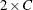or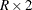.

WTKAP
WTKAPPA

requests an exact test for the weighted kappa coefficient. For more information, see the sections Weighted Kappa Coefficient and Exact Statistics. By default, PROC FREQ computes the weighted kappa coefficient by using Cicchetti-Allison agreement weights. You can request Fleiss-Cohen agreement weights by specifying the AGREE(WT=FC) option in the TABLES statement.

Kappa coefficients are defined only for square two-way tables, where the number of rows equals the number of columns. If your table is not square because some observations have weights of 0, you can specify the ZEROS option in the WEIGHT statement to include these observations in the analysis. For more information, see the section Tables with Zero-Weight Rows or Columns.

Fortables, the weighted kappa coefficient is equivalent to the simple kappa coefficient, and PROC FREQ displays only the simple kappa coefficient.

#### Computation Options

The computation-options specify options for computing exact statistics. You can specify the following computation-options in the EXACT statement after a slash (/).

ALPHA=specifies the level of the confidence limits for Monte Carlo p-value estimates. The value ofmust be between 0 and 1; a confidence level ofproduces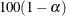% confidence limits. By default ALPHA=0.01, which produces 99% confidence limits for the Monte Carlo estimates.

The ALPHA= option invokes the MC option.

MAXTIME=value

specifies the maximum clock time (in seconds) that PROC FREQ can use to compute an exact p-value. If the procedure does not complete the computation within the specified time, the computation terminates. The MAXTIME= value must be a positive number. This option is available for Monte Carlo estimation of exact p-values, in addition to direct exact p-value computation. For more information, see the section Computational Resources.

MC

requests Monte Carlo estimation of exact p-values instead of direct exact p-value computation. Monte Carlo estimation can be useful for large problems that require a considerable amount of time and memory for exact computations but for which asymptotic approximations might not be sufficient. For more information, see the section Monte Carlo Estimation.

This option is available for all EXACT statistic-options except the BINOMIAL option and the following options that apply only toortables: BARNARD , COMOR , EQOR , MCNEM , OR , RELRISK , and RISKDIFF . PROC FREQ always computes exact tests or confidence limits (not Monte Carlo estimates) for these statistics.

The ALPHA= , N= , and SEED= options invoke the MC option.

MIDP

requests exact mid p-values for the exact tests. The exact mid p-value is defined as the exact p-value minus half the exact point probability. For more information, see the section Definition of p-Values.

The MIDP option is available for all EXACT statement statistic-options except the following: BARNARD , EQOR , OR , RELRISK , and RISKDIFF . You cannot specify both the MIDP option and the MC option.

N=n

specifies the number of samples for Monte Carlo estimation. The value of n must be a positive integer, and the default is 10,000. Larger values of n produce more precise estimates of exact p-values. Because larger values of n generate more samples, the computation time increases.

The N= option invokes the MC option.

PFORMAT=format-name | EXACT

specifies the display format for exact p-values. PROC FREQ applies this format to one- and two-sided exact p-values, exact point probabilities, and exact mid p-values. By default, PROC FREQ displays exact p-values in the PVALUE6.4 format.

You can provide a format-name or you can specify PFORMAT=EXACT to control the format of exact p-values. The value of format-name can be any standard SAS numeric format or a user-defined format. The format length must not exceed 24. For information about formats, see the FORMAT procedure in the Base SAS Procedures Guide and the FORMAT statement and SAS format in SAS Formats and Informats: Reference.

If you specify PFORMAT=EXACT, PROC FREQ uses the 6.4 format to display exact p-values that are greater than or equal to 0.001; the procedure uses the E10.3 format to display values that are between 0.000 and 0.001. This is the format that PROC FREQ uses to display exact p-values in releases before SAS/STAT 12.3. Beginning in SAS/STAT 12.3, by default PROC FREQ uses the PVALUE6.4 format to display exact p-values.

POINT

requests exact point probabilities for the exact tests. The exact point probability is the exact probability that the test statistic equals the observed value. For more information, see the section Definition of p-Values.

The POINT option is available for all EXACT statement statistic-options except the following: BARNARD , EQOR , OR , RELRISK , and RISKDIFF . You cannot specify both the POINT option and the MC option.

SEED=number

specifies the initial seed for random number generation for Monte Carlo estimation. The value of the SEED= option must be an integer. If you do not specify the SEED= option or if the SEED= value is negative or 0, PROC FREQ uses the time of day from the computer’s clock to obtain the initial seed.

The SEED= option invokes the MC option.